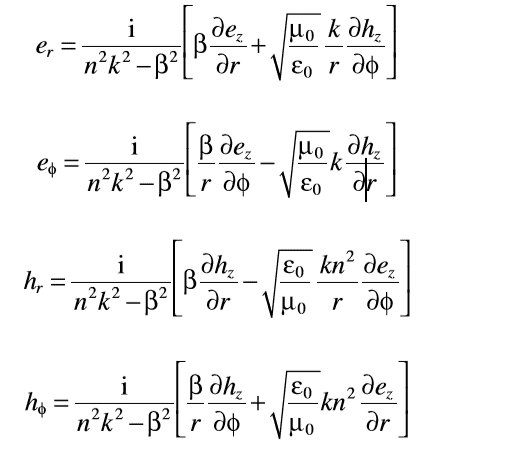# ∇ x E in Cylindrical Cordinates

## Homework Statement

I would like to re-write equation according to polar coordinates. ∇ x E = determinant of Polar coordinates. My question is how can i write determinant of polar coordinates?

## The Attempt at a Solution

E(x,y,z)= e(x,y) e^jβz
first e is a vector. ^ means exponantial

x=r.cos(φ)
y=r.sin(φ)

polar coordinates including e(r),e(φ),e(z)

is it true?

1. row i j k
2. row d/dr d/dφ d/dz
3. row e(r) e(φ) e(z)

so where can i use
x=r.cos(φ)
y=r.sin(φ)

Staff Emeritus
Homework Helper
Gold Member
2021 Award
No. That is not the appropriate form for the curl in cylinder coordinates. You should be able to find the appropriate expression by a simple obline search for ”curl in cylinder coordinates”

•İf possible, could you look a book named by Fiber Lasers, Basics Tech and App's. In this book page 12, you will see the equations set in polar coordinates for Maxwell equations.

Maxwell Equations set in polar coordinates:My homework is by using Maxwell equations in polar coordinates, to reach this Picture. But i did, what you said. I wrote the determinant. But i could'nt find. Could you use maxwell equations ∇ x E and ∇ x H till this Picture, step by step.

Thanks!

#### Attachments

Last edited:
can someone help?

Staff Emeritus
This entire thread is VERY confusing.

The title of the thread asked about the curl of E in cylindrical coordinate, which should be trivial to write (look it up!). But then, the body of the thread is asking about polar coordinates and writing its determinant?!! It is cylindrical, or is it polar?

And are you asking how for cylindrical coordinates, or spherical polar coordinates? Make up your mind.

And forget about taking the curl of anything. Do you know how to use the determinant method to find the cross product of two vectors in the first place?

BTW, just for reference, this is all math, not physics.

Zz.

•can someone help?

in cylindrical coordinates not polar. just book says its in polar coordinates

Homework Helper
Gold Member
2021 Award
•$$\boldsymbol{\nabla}=\mathbf{i_r}\frac{\partial }{\partial r}+\mathbf{i_{\theta}}\frac{1}{r}\frac{\partial }{\partial \theta}+\mathbf{i_z}\frac{\partial }{\partial z}$$and
$$\mathbf{E}=E_r\mathbf{i_r}+E_{\theta}\mathbf{i_{\theta}}+E_z\mathbf{i_z}$$
So, $$\boldsymbol{\nabla}\boldsymbol{\times}\mathbf{E}=\mathbf{i_r}\boldsymbol{\times}\frac{\partial \mathbf{E}}{\partial r}+\mathbf{i_{\theta}}\boldsymbol{\times}\frac{1}{r}\frac{\partial \mathbf{E}}{\partial \theta}+\mathbf{i_z}\boldsymbol{\times}\frac{\partial \mathbf{E}}{\partial z}$$So, what do you get for ##\frac{\partial \mathbf{E}}{\partial r}##, ##\frac{\partial \mathbf{E}}{\partial \theta}##, and ##\frac{\partial \mathbf{E}}{\partial z}##?
•